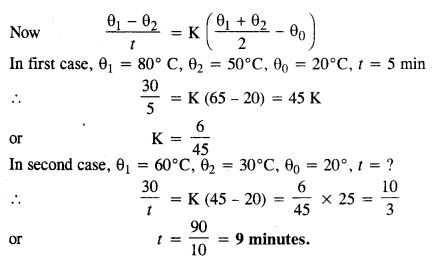## NCERT Solutions for Class 11 Physics Chapter 11 Thermal Properties of Matter

These Solutions are part of NCERT Solutions for Class 11 Physics. Here we have given NCERT Solutions for Class 11 Physics Chapter 11 Thermal Properties of Matter

Question 1.
The triple points of neon and carbon dioxide are 24.57 K and 216.55 K respectively. Express these temperatures on the Celsius and Fahrenheit scales.
The relation between Kelvin scale and Celcius scale is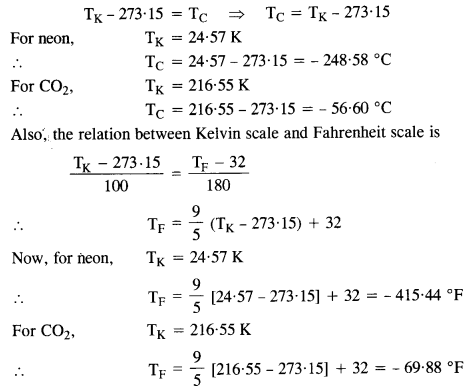Question 2.
Two absolute scales A and B have triple points of water defined to be 200 A and 350 B. What is the relation between TA and TB?
The triple point of water is T = 273.16 K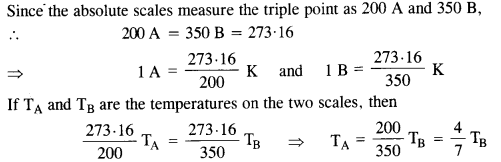Question 3.
The electrical resistance in ohms of a certain thermometer varies with temperature according to the approximate law:
R = R0 [1 + 5 x 10-3 (T – T0)]
The resistance is 101.6 Ω at the triple-point of water, and 165.5 Ω at the normal melting point of lead (600.5 K). What is the temperature when the resistance is 123.4 Ω ?
At the triple point of water, T = 273.16 K and R = 101.6 Ω(given)
R = R[1 + 5 X 10-3  (T – T0)]
=>  101.6 = Ro [1 + 5 X 10-3 (273-16-T0)]  …(1)
At temperature T = 600.5 K, R = 165.5 Q
165.5 = Ro [1 + 5 X 10-3 (600-5 – T0)]……………(2)(a) The triple-point of water is a standard fixed point in modern thermometry. Why? What is wrong with taking the melting point of ice and the boiling point of water as standard fixed points (as was originally done in the Celsius scale)?
(b) There were two fixed points in the original Celsius scale as mentioned above which were assigned the number 0 °C and 100 °C respectively. On the absolute scale, one of the fixed points is the triple-point of water, which on the kelvin absolute scale is assigned the number 273.16 K. What is the other fixed point on this (Kelvin) scale?
(c) The absolute temperature (Kelvin scale) T is related to the temperature tc on the Celsius scale by
tc = T – 273.15
(d) Why do we have 273.15 in this relation, and not 273.16?
What is the temperature of the triple-point of water on an absolute scale whose unit interval size is equal to that of the Fahrenheit scale?
(a) Triple point of water has a unique value e. 273.16 K. The melting point and boiling points of ice, and water respectively do not have unique values and change with the change in pressure.
(b) The other fixed point is absolute zero of temperature.
(c) On the Celcius scale 0 °C corresponds to the melting point of ice at normal pressure and the value of absolute temperature is 273.15 K. The temperature 273.16 K corresponds to the triple point of water.
(d) The Fahrenheit scale and Absolute scale are related as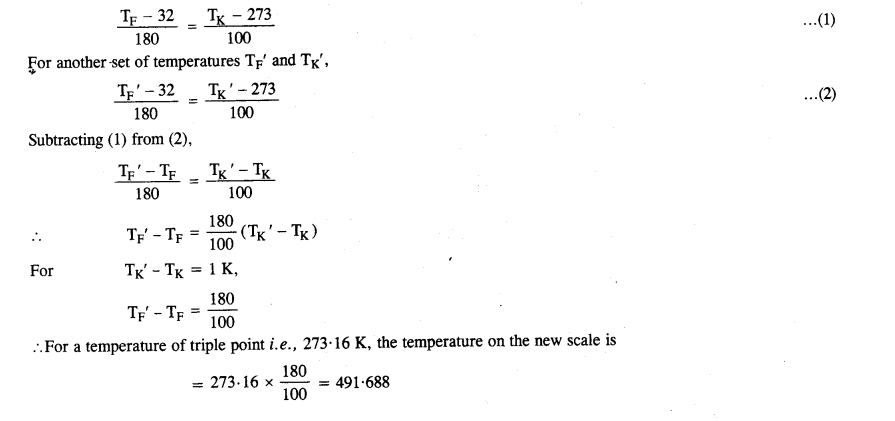Question 5.
Two ideal gas thermometers A and B use oxygen and hydrogen respectively. The following observations are made :

 Temperature Pressure thermometer A Pressure thermometer B Triple-point of water Normal melting point of sulphur 1.250 x 105 Pa 1.797 x 105 Pa 0.200 x 105 Pa 0.287 x 105 Pa

(a) What is the absolute temperature of the normal melting point of sulphur as read by thermometers A and B?
(b) What do you think is the reason for the slightly different answers from A and B? (The thermometers are not faulty). What further procedure is needed in the experiment to reduce the discrepancy between the two readings?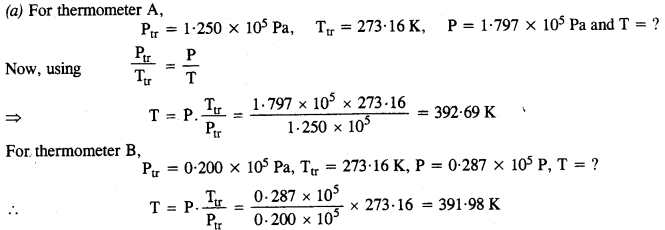(b) The discrepancy between the two readings is due to the fact that the gases are not perfectly ideal gases. To reduce the discrepancy, the readings should be taken at low pressures so that the gases could show prefect behaviour.

Question 6.
A steel tape 1 m long is correctly calibrated for a temperature of 27.0 °C. The length of a steel rod measured by this tape is found to be 63.0 cm on a hot day when the temperature is 45.0 °C. What is the actual length of the steel rod on that day? What is the length of the same steel rod on a day when the temperature is 27.0°C? Coefficient of linear expansion of steel = 1.20 x 10-5 °C_1.
The length of the steel tape at 27 °C is 100 cm
∴  L = 100 m and T = 27°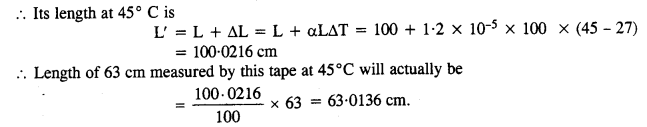Length of the same steel rod shall again be 63 cm when measured by the same steel tape at 27°.

Question 7.
A large steel wheel is to be fitted onto a shaft of the same material. At 27°C, the outer diameter of the shaft is 8.70 cm and the diameter of the central holes in the wheel is 8.69 cm. The shaft is cooled using ‘dry ice’. At what temperature of the shaft does the wheel slip on the shaft? Assume the coefficient of linear expansion of the steel to be constant over the required temperature range.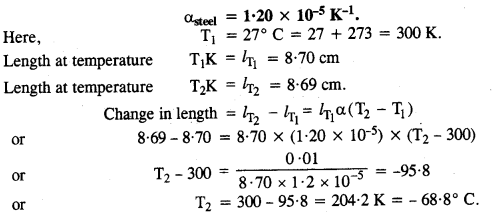Question 8.
A hole is drilled in copper steel. The diameter of the hole is 4.24 cm at 27.0 °C. What is the change in the diameter of the hole when the steel is heated to 227 °C? Coefficient of linear expansion of copper = 1.70 x 10-5 °C1
Given α = 1.70 × 10-5K-1
β = 2α = 3.40 × 10-5K-1
d1 = 4.24 cm
Initial area A1 at 27°C = 300K = T1
is $$\pi\left(\frac{\mathrm{d}_{1}}{2}\right)^{2}=\pi\left(\frac{4.24}{2}\right)^{2}$$
⇒ A1 = 4.4944 π cm2
Area A2 at 227°C = 500K = T2 is
1 [1 + β(T2 – T1)]
i.e. A2 = 4.4944 π [1 + 3.40 × 10-5 (500 – 300)]
A2 = 4.5250 π cm2
⇒ $$\pi\left(\frac{\mathrm{d}_{2}}{2}\right)^{2}$$ = 4.5250 π cm2
⇒ d2 = $$\sqrt{18.100}$$ =4.2544 cm
∴ Change in diameter = d2 – d1
= 4.2544 – 4.25 = 0.0144 cm

Question 9.
A brass wire 1.8 m long at 27°C is held taut with little tension between two rigid supports. If the wire is cooled to a temperature of – 39 °C, what is the tension developed in the wire, if its diameter is 2.0 mm? Co-efficient of linear expansion of brass = 2.0 x 10-5 °C’ ; Young’s modulus of brass = 0.91 x 1011 Pa.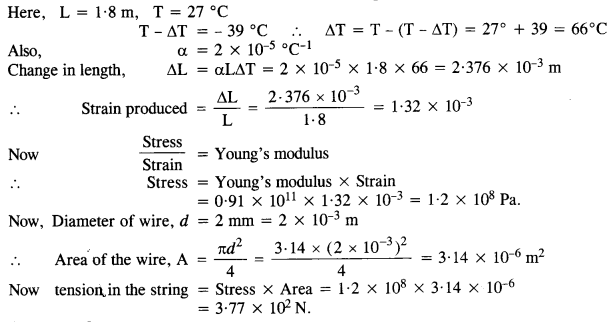Question 10.
A brass rod of length 50 cm and diameter 3.0 mm is joined to a steel rod of the same length and diameter. What is the change in length of the combined rod at 250 °C, if the original lengths are at 40*0 °C? Is there a ‘thermal stress’ developed at the junction? The ends of the rod are free to expand (Co-efficient of linear expansion of brass = 2.0 x 10-5 °C1, steel = 1.2 x 105 °C1.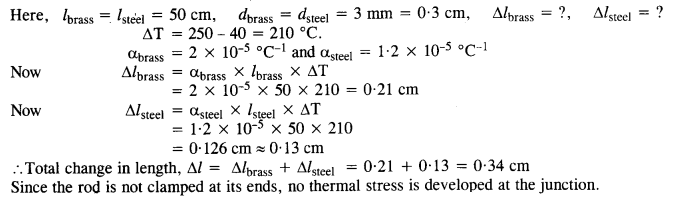Question 11.
The coefficient of volume expansion of glycerin is 49 x 10-5 °C-1. What is the fractional change in its density for a 30 °C rise in temperature?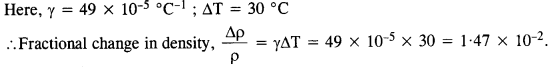Question 12.
A 10 kW drilling machine is used to drill a bore in a small aluminium block of mass 8.0 kg. How much is the rise in temperature of the block in 2.5 minutes, assuming 50% of power is used up in beating the machine itself or lost to the surroundings? Specific heat of aluminium = 0.91Jg-1°C-1.Question 13.
A copper block of mass 2.5 kg is heated in a furnace to a temperature of 500°C and then placed on a large ice block. What is the maximum amount of ice that cari melt?
(Specific heat of copper = 0.39J g-1 °C-1, and heat of fusion of water = 335 J g-1).
Let m be the mass of ice melted when hot copper block is placed over an ice block.
Heat lost of copper block = Heat gained by ice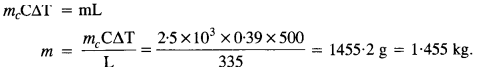Question 14.
In an experiment on the specific heat of a metal, a 0.20 kg block of metal at 150°C is dropped in a copper calorimeter (of water equivalent 0.025 kg) containing 150 cm3 of water at 27°C. The final temperature is 40°C. Compute the specific heat of the metal.Question 15.
Given below are observations on molar-specific heats at room temperature of some common gases.

 Gas Molar specific heat (Cv) (cal mol-1 K-1) Hydrogen 4.87 Nitrogen 4.97 Oxygen 5.02 Nitric oxide 4.99 Carbon monoxide 5.01 Chlorine 6.17

The measured molar specific heats of these gases are markedly different from those for monoatomic gases. [Typically, the molar specific heat of a monoatomic gas is 2.92 cal/mol K, as you must have worked out in 16.] Explain this difference. What can you infer from the somewhat larger (than the rest) value for chlorine?
All the gases mentioned above are diatomic, and hence have other degrees of freedom in addition to the translational degrees of freedom. To raise the temperature of the gas, heat has to be supplied to increase the average energy of all the modes.

Consequently, the molar specific heats for diatomic gases is higher than that for monoatomic gases. The higher value of the molar specific heat of chlorine indicates that at room temperature, vibrational modes are also present In addition to the rotational modes of freedom.

Question 16.
Answer the following questions based on the P – T phase diagram of carbon dioxide (Figure shown below) :(a) At what temperature and pressure can the solid, liquid and vapour phase of CO2 co-exist in equilibrium ?
(b) What is the effect of decrease of pressure on the fusion and boiling point of CO2 ?
(c) What are the critical temperature and pressure for CO2 ? What is their significance ?
(d) Is CO2 solid, liquid or gas at

(a) – 70 °C under 1 atm,
(b) – 60 °C under 10 atm
(c) 15 °C under 56 atm ?

(a) Liquid and vapour phases coexist with solid phase at the triple point temperature – 56.6 °C and a pressure of 5.11 atm.
(b) The boiling point as well as freezing point decrease with the decrease in pressure.
(c) The critical temperature is 3T1 °C and critical pressure is’73.0 atm.
(d) (a) It is vapour (b) It is solid and (c) It is liquid.

Question 17.
Answer the following questions based on the P – T phase diagram of CO2 (Fig. of question 17).
(a) CO2 at 1 atm pressure and temperature – 60 °C is compressed isothermally. Does it go through a liquid phase?
(b) What happens when CO2 at 4 atm pressure is cooled from room temperature at constant pressure?
(c) Describe qualitatively the changes in a given mass of solid CO2 at 10 atm pressure and temperature – 65 °C as it is heated up at room temperature at constant pressure.
(d) CO2 is heated to a temperature of 70° C and compressed isothermally. What changes in its properties do you expect to observe?

1. When subjected to isothermal compression, Co2 will condense to solid directly without passing through the liquid phase.
2. When cooled at constant pressure, Co2 will condense directly to solid phase without passing through the liquid phase,
3. As the solid Co2 is heated up, it first turns to liquid phase and then solid phase. The fusion and boiling points can be obtained from finding out the points of intersection between the horizontal 10 atm line on the P-T diagram with the fusion and vaporization curves.
4. Co2 will not exhibit any clear transition to the liquid phase but will deviate more and more from ideal behaviour ps the pressure increases.

Question 18.
A child running a temperature of 101 °F is given an antipyrin (i. e. a medicine that lowers fever) which causes an increase in the rate of evaporation of sweat from his body. If the fever is brought down to 98 °F in 20 min, what is the average Irate of extra evaporation caused by the drug? Assume the evaporation mechanism to be the only way by which heat is lost. The mass of the child is 30 kg. The specific heat of the human body is approximately the same as that of water, and the latent heat of evaporation of water at that temperature is about 580 cal g_1.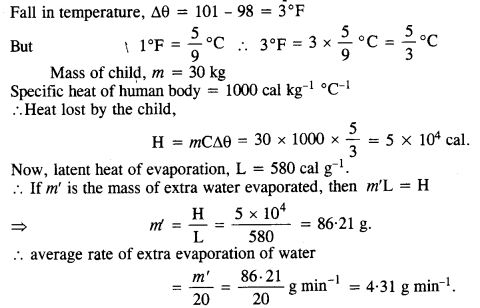Question 19.
A cubical icebox of thermocouples has each side = 30 cm and a thickness of 5 cm. 4 kg of ice is put in the box. If the outside temp is 45° C and coeff. of thermal conductivity = 0.01 Js-1 mf1 °C-1, calculate the mass of ice left after 6 hours. Take latent heat of fusion of ice = 335 x 103 J/kg.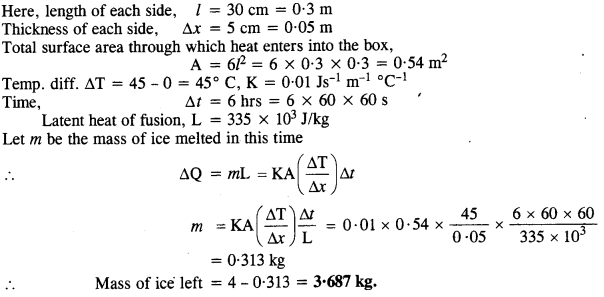Question 20.
A brass boiler has a base area of 0.15 m2 and a thickness of 1.0 cm. It boils water at the rate of 6.0 kg/min when placed on a gas stove. Estimate the temperature of the part of the flame in contact with the boiler. Thermal conductivity of brass = 609 Js-1 m-1 °C-1. Heat of vaporisation of water = 2256 Jg-1.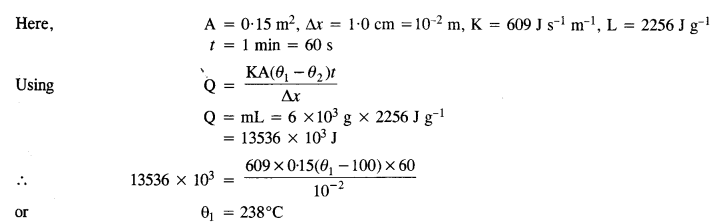Question 21.
Explain why :
(a) a body with large reflectivity is a poor emitter
(b) a brass tumbler feels much colder than a wooden tray on a chilly day
(c) an optical pyrometer (for measuring high temperatures) calibrated for ideal black body radiation gives too low a value for the temperature of a read hot iron piece in the open, but gives a correct value for the temperature when the same piece is in the furnace
(d) the earth without its atmosphere would be inhospitably cold
(e) Heat systems based on the circulation of steam are more efficient in warming a building than those based on circu|ation of hot water.
(a) According to Kirchhoff s law of black body radiations, good emitters are good absorbers and bad emitters are bad absorbers. A body with large reflectivity is a poor absorber of heat and consequently, it is also a poor emitter.

(b) Brass is a good conductor of heat, while wood is a bad conductor. When we touch the brass tumbler on a chilly day, heat starts flowing from our body to the tumbler and we feel it cold. However, when the wooden tray is touched, heat does mot flow from our hands to the tray and we do not feel cold.

(c) An optical pyrometer is based on the principle that the brightness of a glowing surface of a body depends upon its temperature. Therefore, if the temperature of the body is less than 600°C, the image formed by the optical pyrometer is not brilliant and we do not get a reliable result. It is for this reason that the pyrometer gives a too low value for the temperature of red hot iron in the open.

(d) The atmosphere of earth acts as an insulator to stop the infrared radiations which fall on the surface of earth during day time, to escape during the night. If there were no atmosphere, the whole of heat radiated by the earth during night would escape to the universe leaving it under the intense cold.

(e) Steam at a temperature of 100°C has more heat (because its latent heat is 540 cal g-1) compared to the same amount of water at the same temperature. Therefore, heating systems based on the circulation of steam are more efficient in warming a building than those based on the circulation of hot water.

Question 22.
A body cools from 80°C to 50°C in 5 minutes. Calculate the time it takes to cool from 60°C to 30°C. The temperature of the surroundings is 20°C.